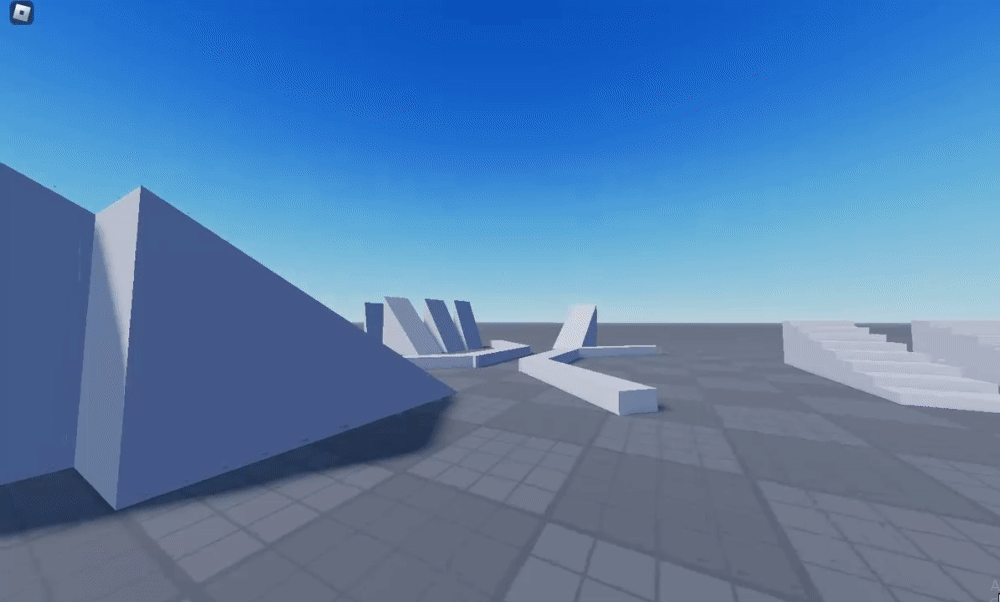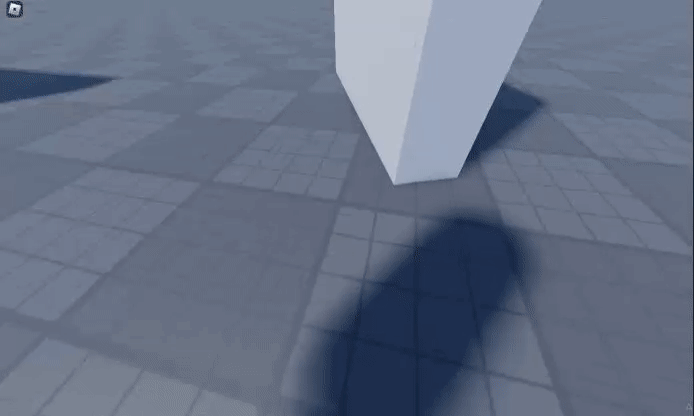# Player drags down wall when they collide with it, rather than slide down itI have made my own character system from scratch. Mostly all the movement mechanics work well (movement momentum, taking stun when falling, etc). I have run into this one problem though. When colliding with walls midair, the player sticks to the wall until their momentum is cancelled, and then they fall.

The system utilizes a Vector3Value object that is manipulated via various things like movement direction, movement speed, building momentum, etc. and then the character’s velocity is then set to that Vector3Value. The problem is, the script doesn’t know to take collisions into account when calculating the velocity, so the player is just continuously rammed into the wall until they slide back down it.I attempted adding a detector to the player to look for parts directly beside the player, and if they were found, the script would not be able to add velocity to the player. While this technically did solve the problem and the player was able to slide down the wall normally, this also killed movement in any direction entirely, both when the player hits the wall and when they land on the ground. I suppose I could also only have it do this while they are also not touching the ground, but this would still make the player immobile until they reached the ground, which is not ideal.

Default Roblox characters seem to interact with walls fine without any trouble, I’d love to know what they’re doing that I am missing.

I would suggest adding velocity onto the player’s current velocity instead of setting the player’s velocity.

I have attempted this also, The player still inexplicably drags down the wall.

The only thing left (though extremely long winded) would be to detect the wall. If the wall is detected, use some wild means of raycasting to get a Vector3 velocity value that is perpendicular to the normal of the collided object. I have absolutely no idea how I would go about doing this however; and if I were able to pull it off, i fear that the amount of calculation it would take may generate a significant amount of lag.

Maybe, before adding velocity you can raycast from the player’s current position to the position after adding the velocity;

``````local Collision = workspace:Raycast(Player.Position, Velocity)
``````

Then, check if there is a collision, and add gravity onto the velocity before adding it to the player?

``````if Collision then
Velocity += Vector3.yAxis * workspace.Gravity
end

Player.AssemblyLinearVelocity += Velocity
``````

Actually, maybe you can try adding gravity onto the player’s velocity regardless if there is a collision, the player is likely only falling because the physics system is adding gravity onto the player the 3 frames you aren’t changing their velocity (1 game frame = 4 physics frames, physics run 240 times a second).

I have proceeded and done this. I have a block of code below that raycasts in a circle around the player and returns a CFrame value that is the player’s velocity mirrored along the surface of the wall, so that the two values can be interpolated, producing a singular force moving along the surface of the wall.

The function also returns a CFrame pointing opposite the direction of the wall, and a number value “force” (from 0 - 1) which represents how much force should be applied away from the wall depending on how far in the player is pointing into the wall. (i.e. if the player is moving directly at the wall, it will return 1, but if the player is approaching the wall at a slight angle, it will return 0.1 or something)

There are probably plenty of things about this I could clean up, but here’s the function as-is if anybody in the future needs it. I’ve commented it best I could, but even so I wish you the best of luck navigating it.

``````    --   RadialDetect is a function that circles rays about the player in all directions until it locates a wall, returns the direction of the surface
local charPos = PhysicsRoot.Position
local moveDir = CFrame.new(charPos, charPos + moveDir2)

--loop as many times as stated
for i = 1, detail do
--Create a ray along the designated interval
local degrees = ((i-1)/detail ) * 360
local RayCF = CFrame.fromOrientation(0, math.rad(degrees), 0) + charPos

local raycast = Ray.new(charPos, RayCF.LookVector * (PhysicsRoot.Size.X/2 + 0.01) )

--look for parts
local found = false
for _, k in pairs(GetAllParts(raycast, Char)) do
if k.Parent ~= Char and k.CanCollide then
found = k
end
end

--found a part
if found then
--Determine which direction is closer to the direction the player is moving in
local px, py, pz = moveDir:ToOrientation()
local y1 = degrees + 90
local y2 = degrees - 90

--Determine the angle away from the wall (for use later)
local away = degrees - 180
if away < 0 then away = away + 360 end

--format angle into its positive variant
if py < 0 then
end

--If player was moving more left, send them that way. Otherwise send them right
if math.abs(y1 - math.deg(py)) <= math.abs(y2 - math.deg(py)) then
--d1 is the reflected angle
local d1 = 2 * math.rad(y1) - py

--force determines how strong the push away from the wall should be
local force = math.sin(py - math.rad(y1))
--check for NaN errors
if force ~= force then force = nil end

--If d1 sends them into the wall, return nil so their movement is not affected
if py < d1 then
return CFrame.fromOrientation(0, d1, 0), away, force
else
return nil, away, force
end

else
--d1 is the reflected angle
local d1 = 2 * math.rad(y2) - py
if y2 < 0 then
y2 = y2 + 360
end

--force determines how strong the push away from the wall should be
local force = math.sin(py - math.rad(y2))
--check for NaN errors
if force ~= force then force = nil end

--if d1 sends them into the wall, return nil so their movement is not affected
if py > d1 then
return CFrame.fromOrientation(0, d1, 0), away, force
else
return nil, away, force
end
end
end
end

--nothing was found, dont change their movement
return nil

end
``````

This method has produced the following result:Its not perfect but at this point I think I can live with that.

This topic was automatically closed 14 days after the last reply. New replies are no longer allowed.